# Lagrange mean value theorem

(diff) ← Older revision | Latest revision (diff) | Newer revision → (diff)

## Statement

Suppose$f$ is a function defined on a closed interval$[a,b]$ (with$a < b$) such that the following two conditions hold:

1.$f$ is a continuous function on the closed interval$[a,b]$ (i.e., it is right continuous at$a$, left continuous at$b$, and two-sided continuous at all points in the open interval$(a,b)$).
2.$f$ is a differentiable function on the open interval$(a,b)$, i.e., the derivative exists at all points in$(a,b)$. Note that we do not require the derivative of$f$ to be a continuous function.

Then, there exists$c$ in the open interval$(a,b)$ such that the derivative of$f$ at$c$ equals the difference quotient$\Delta f(a,b)$. More explicitly: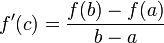$f'(c) = \frac{f(b) - f(a)}{b - a}$

Geometrically, this is equivalent to stating that the tangent line to the graph of$f$ at$c$ is parallel to the chord joining the points$(a,f(a))$ and$(b,f(b))$.

Note that the theorem simply guarantees the existence of$c$, and does not give a formula for finding such a$c$ (which may or may not be unique).

## Proof

Step no. Assertion/construction Facts used Given data used Previous steps used Explanation
1 Consider the function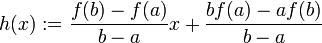$h(x) := \frac{f(b) - f(a)}{b - a} x +\frac{bf(a) - af(b)}{b - a}$.
Then,$h$ is a linear (and hence a continuous and differentiable) function with$h(a) = f(a)$ and$h(b) = f(b)$
Just plug in and check. Secretly, we obtained$h$ by trying to write the equation of the line joining the points$(a,f(a))$ and$(b,f(b))$.
2 Define$g = f - h$ on$[a,b]$, i.e.,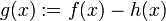$g(x) := f(x) - h(x)$.
3$g$ is continuous on$[a,b]$ Fact (1)$f$ is continuous on$[a,b]$ Steps (1), (2) [SHOW MORE]
4$g$ is differentiable on$(a,b)$ Fact (2)$f$ is differentiable on$(a,b)$ Steps (1), (2) [SHOW MORE]
5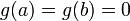$g(a) = g(b) = 0$ Steps (1), (2) [SHOW MORE]
6 There exists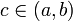$c \in (a,b)$ such that$\!g'(c) = 0$. Fact (3) Steps (3), (4), (5) [SHOW MORE]
7 For the$c$ obtained in step (6),$\! f'(c) = h'(c)$ Fact (4) Steps (2), (6) [SHOW MORE]
8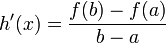$h'(x) = \frac{f(b) - f(a)}{b - a}$ for all$x$. In particular,$h'(c) = \frac{f(b) - f(a)}{b - a}$. Step (1) Differentiate the expression for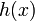$h(x)$ from Step (1).
9$f'(c) = \frac{f(b) - f(a)}{b - a}$ Steps (7), (8) Step-combination direct